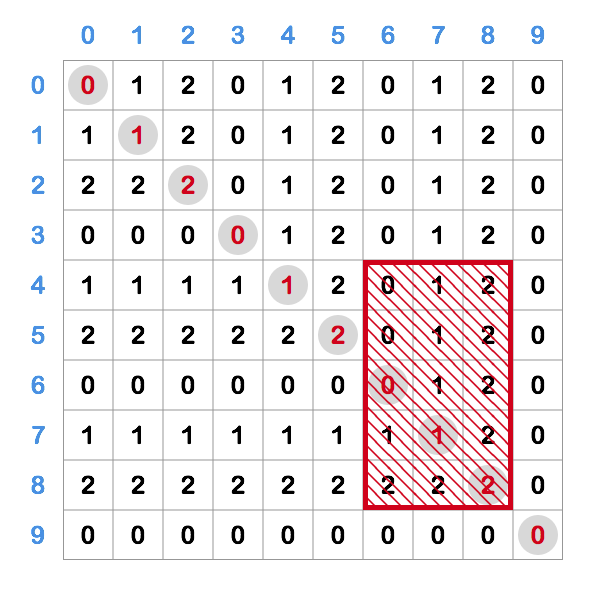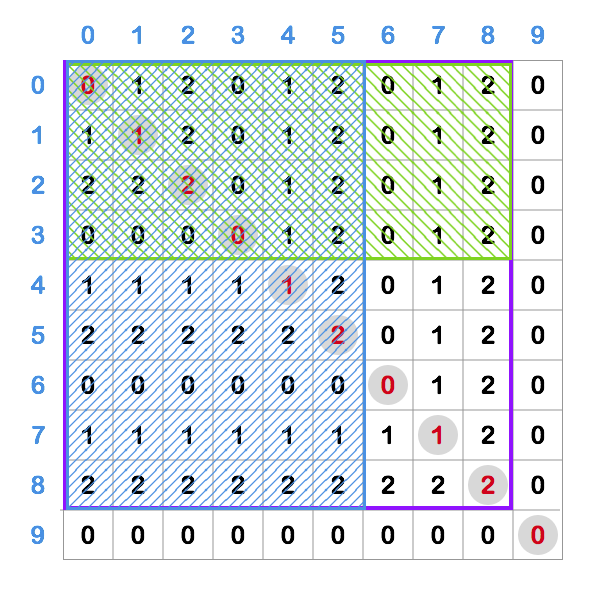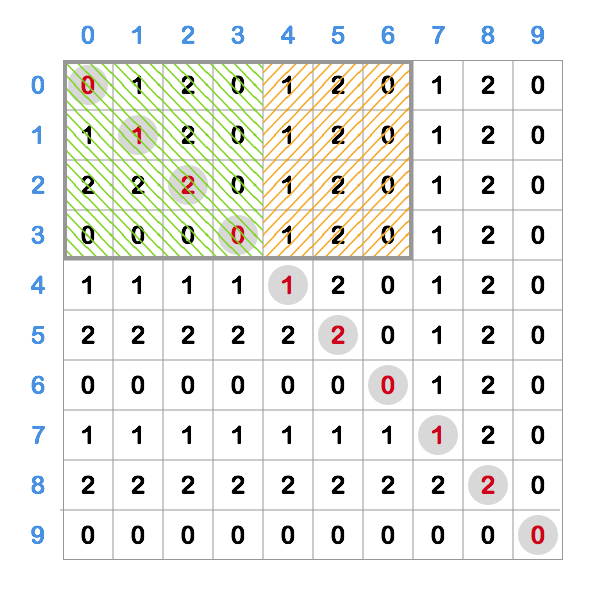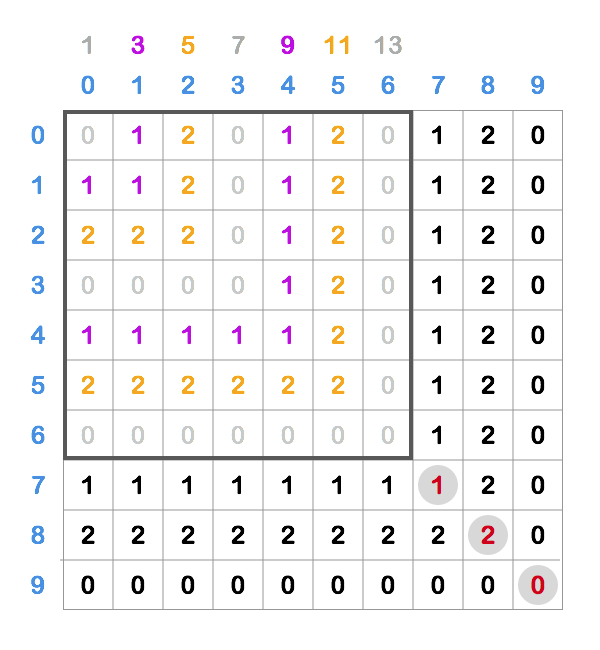# SRM 744 Div II - C++ vs Haskell

SRM 744 was held on December 14th, 2018. The original editorial can be found here. I am currently learning Haskell so I decided to solve all tasks in it as well and then compare to my original C++ solutions.

## ThreePartSplit - Div 2 Easy

Short problem statement: We are given a half open interval `[a, d)` which contains `n = d - a` elements. We need to divide it into three parts such each of them has at least `n div 3` elements. We need to return the “middle” interval as `[b, c)` (so half open again).

Solution: A simple and quick task that can be solved analytically, we just need to be careful not to mess up +-1 indices. There also multiple possible solutions, but maybe the most straightforward one (at least for me) was to assign `n div 3` elements to the first two parts and then whatever is left to the third part.

E.g. if we were given `[0, 8)` as a starting interval, that means we have numbers `0, 1, 2, 3, 4, 5, 6, 7`. If we divided them as described above, we’d end up with intervals `[0, 1], [2, 3], [4, 5, 6, 7]` where we “crammed” all the extra stuff into the last interval.

There are also other valid solutions, e.g. we could’ve put 3 elements in each interval. So if you are using some editor plugin which compares against the example test cases (like Vimcoder) don’t worry if it reports some of your solutions as wrong.

### C++

It was really cool for me to learn here how we can construct and return vector in a single line, very clean and readable.

### Comparison

Language Lines 100 runs
C++ 8 TBD

Although Haskell solutions has a bit less lines than C++ one (mostly due to C++ `class` plumbing), I would say expresiveness-wise solutions are the same. Since it is O(1) analytical solution, we don’t need any looping or mapping or data structures, and with this concise way of creating a vector C++ solution is also very nice to read.

## MagicNumbersAgain - Div 2 Medium

Short problem statement: Number is magic if it is a perfect square and if its digits alternate in a fashion of greater, smaller, greater, smaller, … (e.g. `1 3 2 17 4 2 3`). Given `[A, B]` interval, find out how many magic numbers it contains.

Solution: The naive solution would be to check for each number between `A` and `B` whether it is a magic number or not. However, due to the constraints (interval can have up to 1010 elements), we can see it would not be fast enough in all the possible cases.

But if we could be a bit smarter and somehow first find all the perfect squares in `[A, B]` and then only examine them instead of every number in the interval, would that be more performant? Sure it would, and since we will need to examine at most 105 squares (since 105 hits the upper range bound when squared), we can be sure it is going to be fast enough.

Finding squares is also not a problem, we can just keep iterating over the roots and generate them until we go over the upper range bound.

### C++

A cool trick I learnt here is to generate all the squares from 1 up to B (or even up to the upper limit) and then later just check if it is in the required range. This frees us of doing the work of figuring out what is the first square in the range. Theoretically speaking it is not optimal since we are doing extra work, but here we can afford it and it keeps the code cleaner and less error-prone.

Unfortunately I haven’t used this trick during the competition and made a mistake in just a situation described above (finding first square >= `A`) and my solution failed. So it is a good lesson for the future.

### Comparison

Language Lines 100 runs
C++ 40 TBD

I really enjoyed writing this Haskell solution - I love how concise is the `count` function and how cleanly it breaks the problem into the subproblems that can be addressed (and tested) separately. I especially like `squares` function - how it starts with an infinite list and then we just “trim” it from the both sides until we get the desired result. This way of thinking makes it much easier for me to reason and thus much harder to introduce “off-1” type of bugs.

What I find as most different compared to C++ solution is the situation where we have to check the digits, and compare each digit with the previous digit. Since we don’t do looping as in C++ we have to in advance construct all the “checks” with all the information needed.

## ModularQuadrant - Div 2 Hard

Short problem statement: We are given an infinite, zero-indexed square grid. Each cell contains `max(row, column) mod 3` value. Given a rectangle in that grid, return the sum of its cells.

Solution: The naive solution would be to go over all the cells in the given rectangle, calculate the values and sum it all together. However, since both width and height of the rectangle can be up to 109, it would be way to slow (we’d need to traverse over 1018 cells in the worst case).

So we need to be smarter and somehow use the properties of this grid to our advantage. Let’s examine the portion of the grid of size 10 x 10:I emphasized the diagonal and the red box is an example of rectangle for which we want to calculate the sum of its cells.

We can notice several things here:

• `0 1 2` pattern repeating itself due to `mod 3`
• The grid is symmetrical in respect to the diagonal (i-th row == i-th column)

My first approach was to calculate sum of each row separately and then sum that. I could calculate the sum of row in `O(1)` (observe the patten in a row - number is repeated until the diagonal and then starts cycling), but we still have 109 rows so that was too slow (one test case that took ~6s kept failing).

So we need to be even smarter than that - if calculating a row in `O(1)` is possible, is there also some kind of a rectangle whose sum we could calculate in `O(1)`?

If we could calculate sum of any rectangle that has upper left corner in `0, 0` fast enough, that would be great, because then we use that to calculate sum of any rectangle in the following way:This image should also illustrate what is going on in the formula above (I apologize for the colored mess). If we want to calculate sum of the red box (in the first image) we start with a big purple rectangle and then from it substract the green and blue one. We can see the only thing that is left is what was original a red rectangle. Also, as blue and green rectangles intersect we’ve subtracted that part twice, so we have to add it once to fix that.

That’s it, we’ve managed to express the area of any rectangle with only the rectangles that start in the `0, 0`, upper left corner. So now we only have to find out how to efficiently calculate sum of such a rectangle (`calcRect00Sum` method from above).

If we look at such a rectangle, we can divide it into two parts - square starting at `(0, 0)` (green) and the rest of it (orange).Calculating a square sum - we can see that numbers are progressing in amount as odd numbers, `1, 3, 5, 7, 9, ...` and sum like that we can calculate in `O(1)` so we can use that. Let’s take a look at a bit bigger example of a square starting at `(0, 0)`:Here we can see that the square consists of “L-shaped” layers (colored in the image) that progress in the amount of cells as odd numbers (`1, 3, 5, 7, ...`), which is shown by numbers above the columns in the image.
We can also see that for a particular value (`0, 1, 2`) that the number of cells in the layer progresses by 6 (e.g. first we have 3 `1`s, than 9, than 15, …) - because of this period of 3, we have to “skip” two other numbers before coming to the next occurrence of the one that is observed.

So the sum of the square in the image above can be calculated as:

``````1
0 * (1 + 7 + 13) + 1 * (3 + 9) + 2 * (5 + 11) = 44
``````

We can generalize that to multiplying each value (`0, 1, 2`) with a sum of series that starts at a particular number, has a step of `6` and has number of elements that is equal to the number of “layers” this value has in the square.

If we can calculate the sum of this series in `O(1)` (and we can, check the `seriesSum` method in the code below), that means this whole calculation is done in `O(1)`, which is exactly what we were aiming for.

Calculating the rest - we see it has a repeating pattern in a row so we can use that to calculate the sum.

### Comparison

Language Lines 100 runs
C++ 52 TBD

Implementing Haskell solution was pretty straightforward to implement from the C++ solution, we already divided the problem nicely into functions so we could just implement them in Haskell.

The Haskell solution is naturally shorter due to more dense syntax, but the logic stayed pretty much the same. The final function in Haskell solution, `rectSum`, I had to rename because `sum` is a method that comes in Haskell’s standard library.

Be the first to comment!

# SRM 742 Div II - C++ vs Haskell

SRM 742 was held on November 28th. Since I was participating and I am also currently learning Haskell, I decided to solve all the problems in Haskell as an exercise and then I found it interesting to compare it with C++ solutions.

## BirthdayCandy - Div 2 Easy

Short problem statement: Elisa can pick one of the N bags, each of them holding S amount of candies. Once she picks a bag, she distributes it between her and her K schoolmates in a circular manner (one to each). Once she cannot make a full circle anymore, she takes the rest for herself. Pick a bag so Elisa gets maximum amount of candy.

Solution: This is a simple task and can be solved mostly analytically, we just have to check for each bag how many candies Elisa gets and take the optimal one. To calculate result (how many candies Elisa gets), for a single bag, we just need `div` and `mod` operations to emulate the described distribution algorithm.

### Comparison

Language Lines 100 runs
C++ 8 TBD

Wow! Haskell solution is really amazing here, and this problem is perfect for it. What is beautiful in Haskell solution is that we don’t have to do any initialization and we can even omit one argument of the function.

## SixteenQueens - Div 2 Medium

Short problem statement: We are given a chessboard 50 x 50 and a K queens are already positioned on it in such a way that no queen is attacking any other queens. Given number S, return any S positions on the board where additional S queens can be placed so the mentioned no-attack rule still holds for all the queens on the board.

Solution: What works here is the simplest greedy algorithm - for each queen that is to be placed on the board findy any valid position - place the queen there and repeat the algorithm until all the queens are placed on the board.

Why does this greedy algorithm work in this case? I am not sure. Chessboard size is 50 x 50 cells and there can be at most 16 queens on the board, so I guess that makes the problem “sparse” enough for this to work. But I haven’t yet figured out a formal explanation.

### Comparison

Language Lines 100 runs
C++ 56 TBD

This problem can also be solved very nicely in Haskell! Of course, here we assumme that there will always exist a solution, otherwise we would need to add extra logic to handle those invalid cases.

What I like is how easily we can express the problem in Haskell and break it down into the smaller pieces.

Be the first to comment!

Real World Haskell is a great book with examples on how to use and apply Haskell to the real world problems. While reading it I put here a breakdown of important points I learn in each chapter, so I can better memorize and return later to it when I need it.

## Chapter 9. I/O case study - a library for searching the filesystem

• Introduces combinators - function that takes other functions as arguments and returns new function

## Chapter 10. Parsing a binary data format

Besides just parsing, this chapter puts even more focus on how to organize code and use higher-order functions to reduce boilerplate and duplication (introduces techniques that monads and functors provide for us).

• Prefer ADS (algebraic data structures) over e.g. tuples for passing the state -> ADS is easier to modify/extend since it does not depend on the internal structure of the data (like tuples do).
• Don’t put constraints when defining a data type, but rather on specific functions that require it. That way we keep our code more general and don’t make unneccessary assumptions.
• Introduces a term of lifting a function - make it work with parametrized types.
• Functor - not only for values “inside” of a container, but also for functions.
• Introduces monad techniques for handling context by implementing them themselves.

## Chapter 11. Testing and quality assurance

Introduces QuickCheck as a testing tool for Haskell. What is cool is that it can generate test cases for us.

• We can define a property for which we want to always hold true - QuickCheck will randomly generate test cases for us
• We can define a model implementation and also test that (e.g. our implementation of sort vs. standard library sort) -> no special syntax, uses the same mechanism as above
• With HPC (Haskell Program Coverage) we can observe test coverage of our code in details and identify the weak spots

## Chapter 12. Barcode recognition

This chapter takes as an example a problem of decoding a barcode from the image, but its main purpose is to introduce new data structures, namely `Data.Array` and `Data.Map`.

Going through the example was a bit lengthy and not so easy to follow in the first reading, but it seems to not be using a lot of new techniques besides using the mentioned data structures.

To summarize:

• Introduces `Data.Array` and `Data.Map`, demonstrates how to use them on the example of recognizing a barcode from the image.

## Chapter 13. Data Structures

• Associative lists `[(key, value)]` vs Map
• `xs ++ ys` has quadratic complexity, introduces difference list `(5++) . (3++)...`
• `Data.Sequence` offers improved performance for lists

Introduces monads formally, explains them on a few exammples:

• `Maybe` monad - helps us avoid “staircasing”, unwraping and matching Maybe values continuously.
• `State` monad - helps us pass around a state (e.g. parsing file, generating random values) without having to do it explicitly.
• Introduced `liftM` method(s) - applies function to the inside of Monad
• Implemented our own Monad - Logger which can log things along doing computations

## Chapter 15. Programming with monads

• Introduces generalised lifting via `ap` (`<*>`), instead of `liftMN`
• Introduced `MonadPlus` typeclass - useful to avoid `case`
• When using `newtype`, we can enable directive `GeneralizedNewtypeDeriving` - to e.g. derive `Monad` typeclass
• Introduced `first` and `second` method for applying functions to pair members
• Introduced multi-param type classes - uh
• “Hiding IO”, making it safe - wrap it in a `newtype`
• Introduced `liftIO` - escape hatch from a monad to another monad
• Define monad’s interface through a type class - separate interface from an implementation
• Introduced `Reader` and `Writer` monad
Be the first to comment!